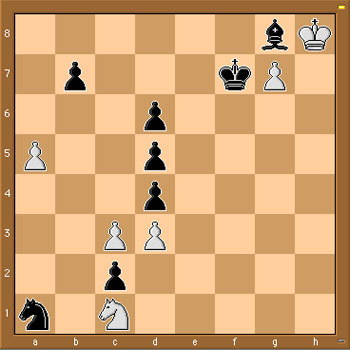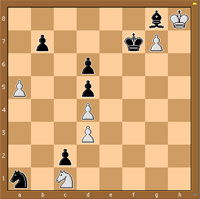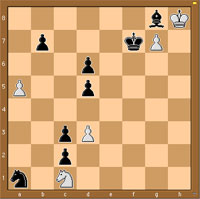Most chess programs are able to give a numerical evaluation of a position. For example, the position below is considered to be worth +8.7 with white to move, and, -0.7 with black to move (by a certain program). But, if one applies combinatorial game theory as in John Conway’s ONAG and the Berlekamp-Conway-Guy masterpiece Winning Ways for your Mathematical Plays it will turn out that the position can be proved to have an infinitesimal advantage for white…So, what do we mean by this? First some basic rules of combinatorial game theory. To start, we evaluate a position without knowing which player has the move. A zero-game is by definition a position in which neither player has a good move, that is, any move by either player quickly leads to losing the game. Hence, a zero-game is a position in which the second player to move wins.

What is the chess-equivalent of a zero-position game? A position in which neither player has a good move is called a Mutual Zugzwang in chess literature. An example is given by the above position, if we restrict attention only to the 4 pieces in the upper right-hand corner and forget the rest. We don’t know who has the move, but, White cannot move at all and Black cannot move the King or Bishop without losing the Bishop and allowing White to promote the pawn and win quickly. In CGT-parlance, the upper-right position has value $\{ \emptyset | \emptyset \} = 0$ where the left options denote the White moves and the right options the Black moves.

All other values are determined by recursion. For example, consider a position in which White has just one move left before the sitution is again a Mutual Zugzwang, and, Black has no good move whatsoever. After white’s move, the position will again be a zero-position and Black has no options, so the value of this position would be denoted by $\{ 0 | \emptyset \}$ and we call the value of this position to be $+1$. Similarly, if white has no options and black has one final move to make, the position would be considered to have value $\{ \emptyset | 0 \}= -1$.

Clearly, these are just the three easiest game-values to have and the real kick comes further down the road when one can prove by recursion that some games have non-integer values (such as $\{ 0 | 1 \} = \frac{1}{2}$ for a position in which white has one move to get to a mutual zugzwang and black has a move leading to a position of value $+1$ (defined as before)), or non-number values such as $\ast = \{ 0 | 0 \}$ where both white and black’s best move is to get to a mutal zugzwang. Game-values such as $\ast$ are called fuzzy (or confused with zero) and are defined by the property that the first player to move wins.

Similarly, positive game-values are those positions where White wins, independent of who has the move and negatives are those that Black wins. There is a whole menagery of game-values and the WinningWays-booklets give an example based introduction to this fascinating theory.

Brief as this introduction was, it will allow us to determine the exact value of the position in the above diagram. We know already that we can forget about the right-hand upper corner (as this is a zero-position) and concentrate attention to the left-hand side of the board.

It is easy to see that neither Knight can move without loosing quickly, nor can the pawns on a5 and b7. That is, white has just 2 options : either c3-c4 (quickly loosing after d5xc4 2. d3xc4,d4-d3 3. Nc1xd3,Na1-b3) or, and this is the only valid option c3xd4 leading to the position on the left below. Black has only one valid move : d4xc3 leading to the position on the right below.Clearly, the left-diagram has value 0 as it is a mutual Zugzwang. The position on the right takes a moment’s thought : White has one move left d3-d4 leading to a 0-position, whereas black has one move d5-d4 leading to a position of value -1 (as black still has one move left d6-d5, whereas white has none). That is, the CGT-value of the right-hand position is $\{ 0 | -1 \}$ and therefore, the value of the starting position is precisely equal to

$\{ 0 | \{ 0 | -1 \} \} = +_{1}$

(called tiny-one among ONAGers)

It can be shown that $+_1$ has a positive value (that is, White wins independently of who has the first move) but smaller than any positive number-valued games!

Noam Elkies has written a beautiful paper On numbers and endgames: Combinatorial game theory in chess endgames containing many interesting examples (the example above is an adaptation of his diagram9).

Published in games